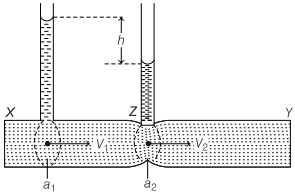We are giving a detailed and clear sheet on all Physics Notes that are very useful to understand the Basic Physics Concepts.

Venturimeter Definition:
It is a device used for measuring the rate of flow of liquid through pipes. Its working is based on Bernoulli’s theorem.
Rate of flow of liquid,

v = a1a2$$\sqrt{\frac{2 g h}{a_{1}^{2}-a_{2}^{2}}}$$where, a1 and a2 are area of cross-sections of tube at broader and narrower part and h is difference of liquid columns in vertical tubes.

• Low losses.
• Suitable for the large diameter of the pipe.
• It can be installed vertically, horizontally, inclined.
• Less chance of getting stuck with sediment.